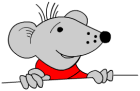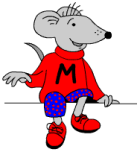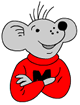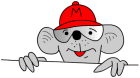## Booster maths worksheet: Time (pg 3)

Being able to read information from a calendar may seem pretty straightforward to us, but many children do not come across these in their everyday life and hence have problems working out how to extract the information.

The questions here are aimed at boosting a level 3 towards a level 4. For those children aiming at a Level 5 care must be taken to read the tables and charts accurately as they are a good way to pick up easy marks.

Booster maths worksheet: Time (pg 3)

## Booster maths worksheet: Time (pg 2)

This free maths worksheet also looks at a.m. and p.m.

Let’s crack on with some more worksheets for practising for the SATs.

This free maths worksheet also looks at a.m. and p.m. The questions have to be read carefully to make sure that the minutes are either added to the time or subtracted.

Booster maths worksheet: Time (pg 2)

## Booster maths worksheet: Time (pg 1)

Time is a popular theme with the test writers and they are pretty keen on testing knowledge of a.m. and p.m. and how the 24 hour clock works. These straightforward questions are of the kind found early in the test and are easy marks to pick up to ensure a Level 4.

Booster maths worksheet: Time (pg 1)

## Free Y5 maths worksheet: Ordering measurements

This is a good free maths worksheet to encourage systematic ordering of data.This is a good free maths worksheet to encourage systematic ordering of data. Lists of highest mountains, longest rivers and largest turtles are given, but they are not in order. There is a definite technique in efficiently putting these in order as it is very easy to miss one and not so easy to rearrange them if you are doing it on paper!

For example, take the mountains. They are all over 8000 m in height, so the key number to look at is the hundreds digit. Find the highest (Everest) and mark a 1 next to it. Find the second highest and mark it with a 2 and so on. Then rewrite them according to the marks.

Some good spin-off work here for Geography – can they find the mountains, rivers etc?

Free Y5 maths worksheet: Ordering measurements

## Free Y4 maths worksheet: Concave and convex shapesWe usually think of the terms convex and concave in relation to mirrors and lens. The word convex means curving out or bulging out but it can also be applied to polygons. A convex polygon is one where all the internal angles are less than 180 degrees – the type that we are most familiar with. A concave polygon will have an interior angle greater than 180 degrees – it looks as if it has been pushed in on one side.

Free Y4 maths worksheet: Concave and convex shapes

## Ordering game: Put the clowns’ balls in order

Drag the numbers on to the yellow balls, in order, starting with the lowest from left to right.

The clowns are trying to sort their balls into order. Help by dragging the numbers on to the yellow balls, in order, starting with the lowest from left to right.

Press Go when finished.

## Free Y3 maths worksheet: Measuring in kilometres

This free maths worksheet concentrates on writing half kilometres as decimal fractions.Children in the UK get far less experience at using kilometres than most Euoropeans because we have decided to keep with the mile for most of our longer measuring. Of course this is a nonsense: to start with a system using mm, cm and metres and then switch to a completely different system ie miles does not make any sense at all! Until our road signs are changed there is little hope of any improvement in this situation.

Whilst there are 1760 yards in a mile the much simpler metric system has the easy to calculate 1000 metres in a kilometre.

At this stage children should be beginning to write half a kilometre as 0.5 km but 1/2 km is acceptable. This free maths worksheet concentrates on writing half kilometres as decimal fractions.

Free Y3 maths worksheet: Measuring in kilometres (pg 3)

## Booster maths worksheet 13 and 14

Two similar maths worksheets here which demonstrate a number of techniques that can be used to answer SAT questions.

The first question asks for two numbers which add up to 140 from the selection given. The easiest approach to this is firstly to look at the units and find two which add up to 10, such as 28 and 32. Then to look to see if the tens digits are suitable; in this case they are not.

Try again 99 and 91 – this time too big.

Try 74 and 66.

6 and 4 make 10. 70 and 60 make 130. Add 10 to 130 makes 140 – there is your answer!

For question 3 the order is important. Start with an edge of the triangle which already has two numbers in.

Booster maths worksheet 14

## Booster maths worksheet 12

Time to crack on with some more Booster worksheets as the dreaded SATs are not far away.I will be concentrating on these maths worksheets for the next couple of weeks. For many children just a few extra marks can raise their level from a 3 to a 4 or a 4 to a 5. I’m not sure what it all means in the wider world but it is certainly good for children’s self confidence if they can get that higher level. The worksheet below is further practice at logical thinking.

Booster maths worksheet 12

## Reception maths vocabulary: Position, direction and movement

Reception maths vocabulary: Position, direction and movement

Words which describe the position and direction of objects are very important to use with your children. Confidence in using these terms enables children to describe what is happening in the world around them. Many of the movement words are later used in mathematics with such topics as shape, co-ordinates, translations etc.

Reception maths vocabulary: Position, direction and movement